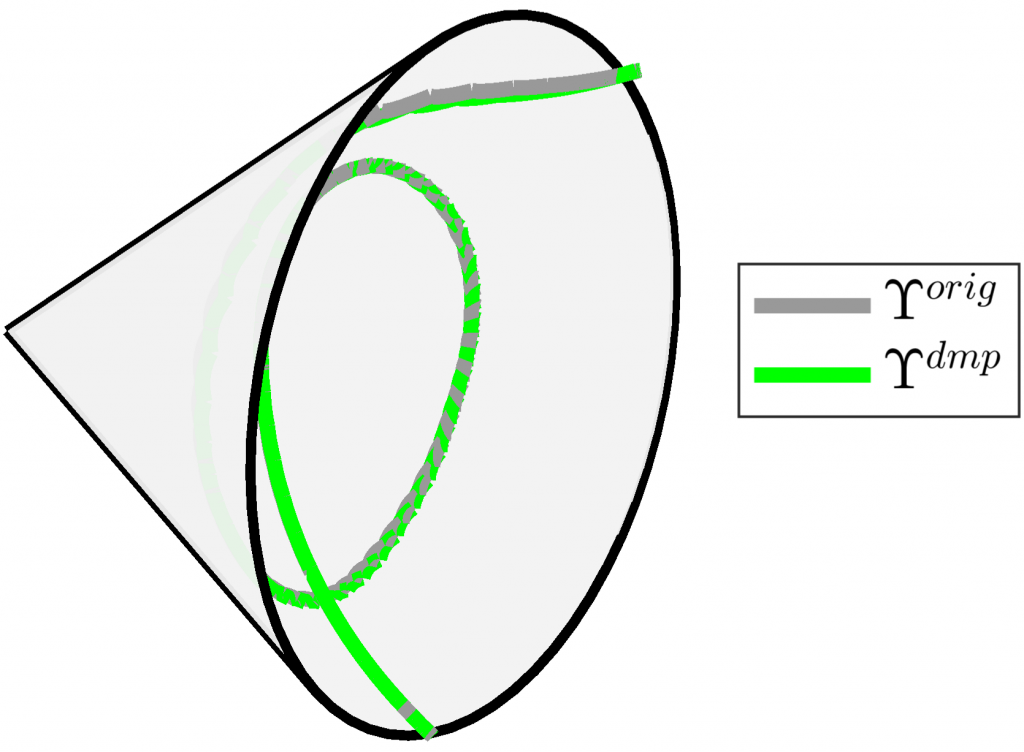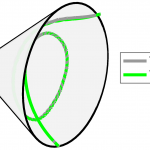# Learning geometry-based robot-manipulation skillsRepresentation of the demonstration (in gray) and reproduced (in green) manipulability ellipsoids in the cone of SPD manifold.

In many robot control problems, skills such as stiffness, damping and manipulability ellipsoids are naturally represented as symmetric positive definite (SPD) matrices, orientations are represented as unit quaternions, sensory data processed as spatial covariances, etc., which capture the specific geometric characteristics of those skills. Typical learned skill models such as dynamic movement primitives (DMPs), probabilistic learning algorithms, kernelized movement primitives, etc., can not, be directly employed with quantities expressed as SPD or unit quaternion as they are limited to data in Euclidean space. These skills demand special treatment using geometric methods based on Riemannian manifolds.
In this line of research, we investigate ways to develop new algorithms capable of learning and adapting geometry-form robot manipulation skills by exploiting metric learning approaches in order to build a Riemannian manifold of skill instances or tasks.

## People involved